# CBSE Class 12 Maths Exam 2018: Question Paper Analysis

The Central Board of Secondary Education which is commonly known as CBSE is one of the oldest and most preferred educational board in India. CBSE conducted the class 12 mathematics examination on 21st March 2018. The duration of the exam was 3 hours with a total marks of 100. Around 5 lakh students appeared for the class 12 mathematics examination this year. The complete question paper analysis of the class 12 mathematics exam is given below.

### Question Pattern

The CBSE Class 12 Maths exam paper consisted of 4 sections namely A, B, C, and D. There were no overall choices available throughout the question paper, however, there was 3 internal choice question of 4 marks each and 3 internal choice question of 6 marks each. The detailed CBSE Class 12 Maths exam pattern is given in the table below.

 Section No. Of Questions Marks of Each Question Total Marks A 4 1 4 B 8 2 16 C 11 4 44 D 6 6 36 Total – 29 100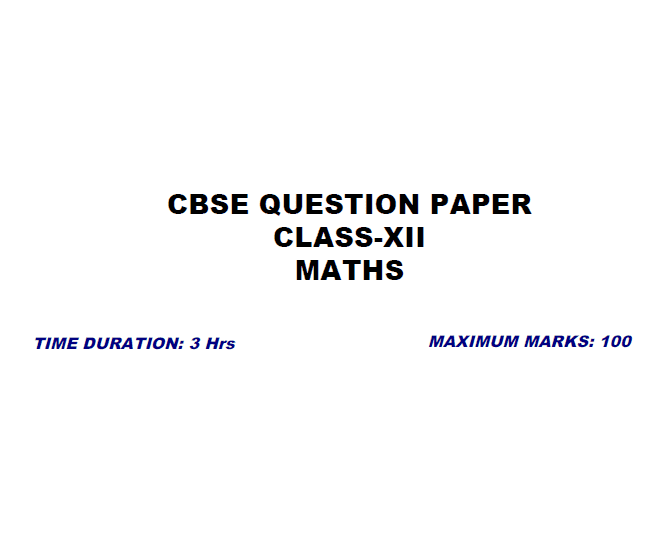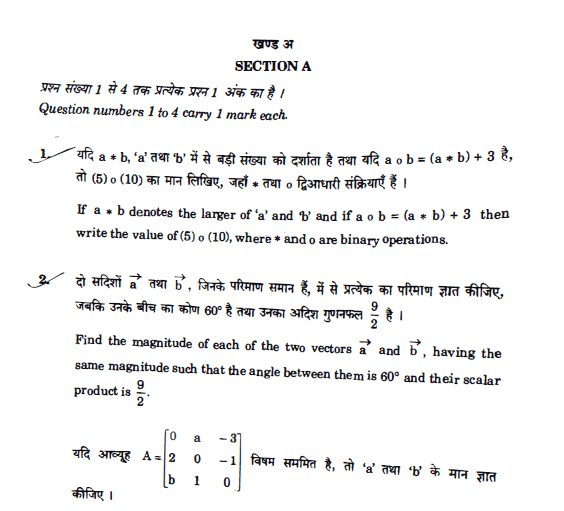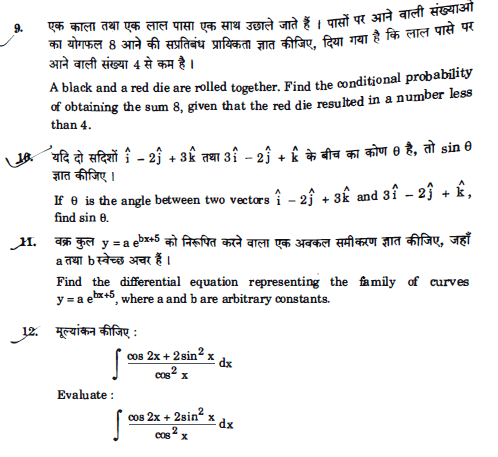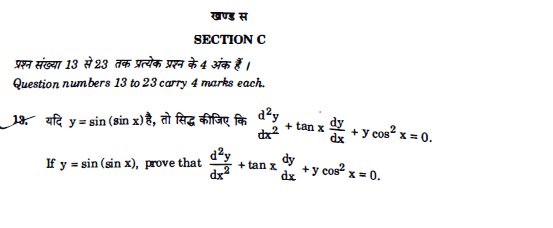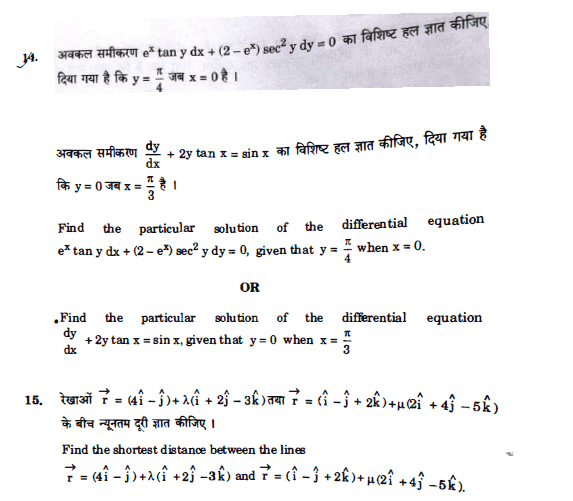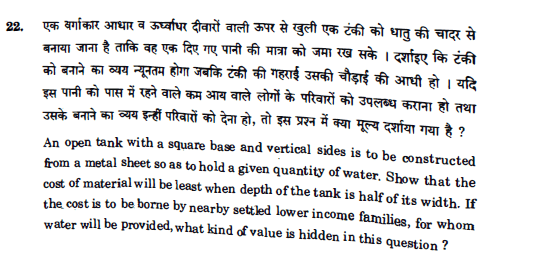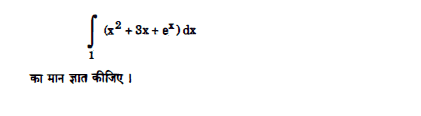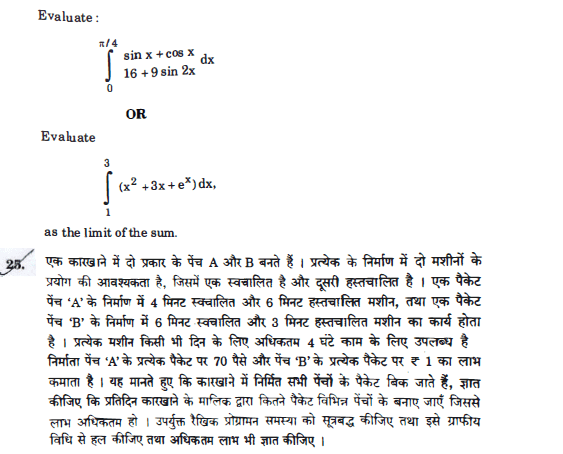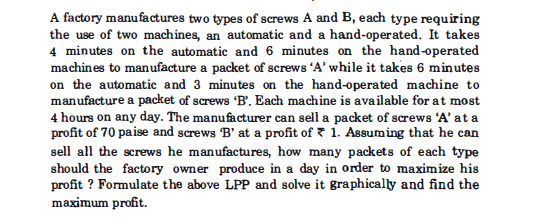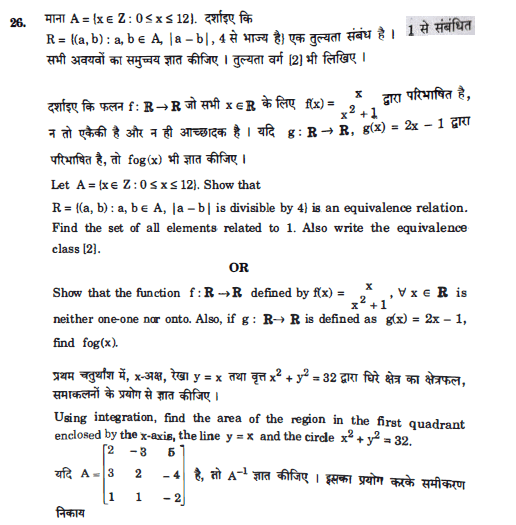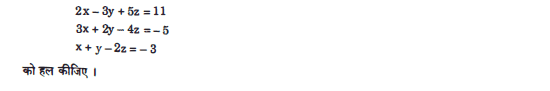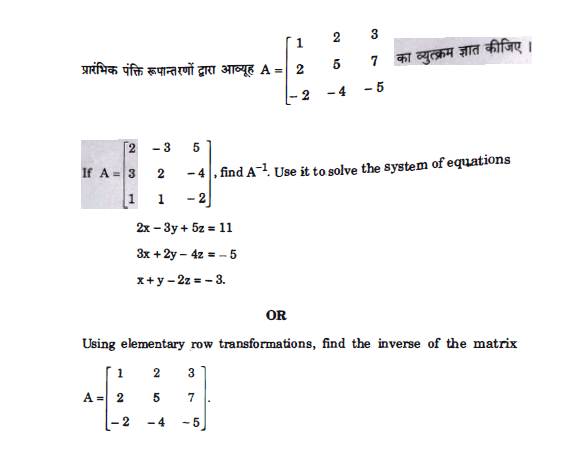### Chapter-Wise Marks Weightage

The weightage of marks was same as that of CBSE 2017 Maths exam. Questions from Differential Equations and 3D Geometry were a lot easier than the previous year. The maximum number of questions came from the chapters Probability, Vector Algebra, and Determinants. All the questions came from the CBSE Class 12 Maths syllabus.

The chapter wise marks weightage along with the comparison with last years paper is given in the table below.

 Topic Name Marks Weightage (2017) Marks Weightage (2018) Relations and Functions 6 1+6=7 Matrices 8 1+2=3 Inverse Trigonometric Functions 4 1+2=3 Continuity and Differentiability 10 2+4=6 Determinants 5 4+6=10 Integrals 10 2+4=6 Application of Derivatives 12 2+4=6 Differential Equations 6 4+2=6 Application of Integrals 6 6 Three Dimensional Geometry 9 4+6=10 Vector Algebra 6 1+2+4=7 Probability 10 4+4+2=10 Linear Programming 6 6

### Overview of CBSE Class 12 Maths Exam 2018

 Difficulty Level Easy Time-Consuming Section Section D Questions Out Of Syllabus No Repeated Questions From Last Year No Average Expected Marks 75 – 90

### General Review

The 2018 Class 12 maths question paper was easier than the previous year. Few questions were similar to that of NCERT Class 12 Maths textbooks. According to exam analyst, 70 to 80 marks are easily scorable in the exam as most of the questions were to NCERT level.

Stay tuned with BYJU’S to get the latest notification on CBSE along with other exam details like date sheet, syllabus, exam pattern, marking scheme, etc.